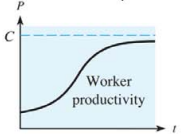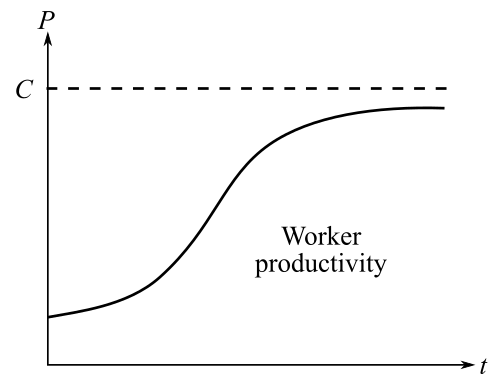Chapter 10.5, Problem 41E### Mathematical Applications for the ...

11th Edition
Ronald J. Harshbarger + 1 other
ISBN: 9781305108042

#### Solutions

Chapter
Section### Mathematical Applications for the ...

11th Edition
Ronald J. Harshbarger + 1 other
ISBN: 9781305108042
Textbook Problem

# Productivity The figure is a typical graph of worker productivity per hour P as a function of time t on the job.(a) What is the horizontal asymptote?(b) What is lim x → ∞ P ( t ) ? (c) What is the horizontal asymptote for P ' ( t ) ? (d) What is lim x → ∞ P ' ( t ) ?(a)

To determine

The horizontal asymptote of the provided graph:Explanation

Given information:

The provided graph is:

Explanation:

An asymptote is a line or axis to which a graph never meets...

(b)

To determine

The value of limxP(t) by from the provided graph.(c)

To determine

The horizontal asymptote of the P(t) using the provided graph:(d)

To determine

The value of limxP(t) by from the provided graph.### Still sussing out bartleby?

Check out a sample textbook solution.

See a sample solution

#### The Solution to Your Study Problems

Bartleby provides explanations to thousands of textbook problems written by our experts, many with advanced degrees!

Get Started

#### Find more solutions based on key concepts